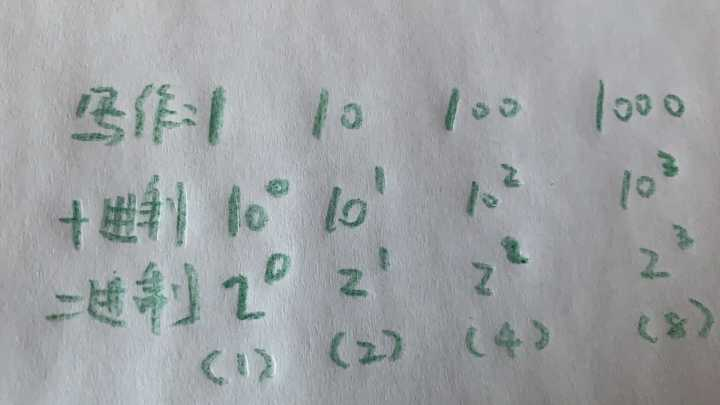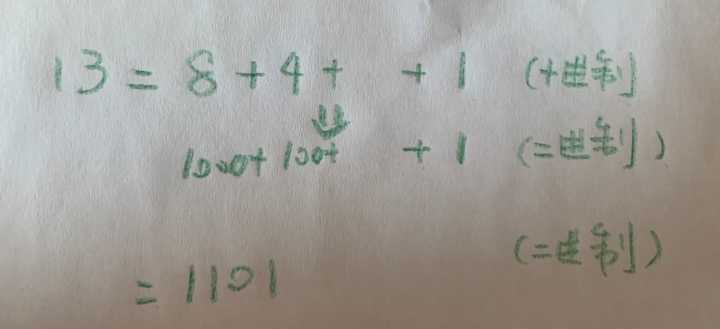# 进制转换的原理是什么？

• 知乎
• 优质文章

123=1×100+2×10+3×1

9876=9×1000+8×100+7×10+6×1

10^0(10的0次方，后同)，10^1,10^2,10^3……（由于二进制中非零的数只有1，所以其实等同于：17=16+1=二进制10001

19=16+2+1=二进制10011

30=16+8+4+2=二进制11110

……

（1）一个二进制数末尾是1，意味着一定是……+1，前面的每个数都是2的倍数，只有最后的+1不是

（2）如果一个二进制数从低往高第2位是1，我们希望把它转换为（1）的情况，那么我们把这个二进制数的末尾抹掉。

……